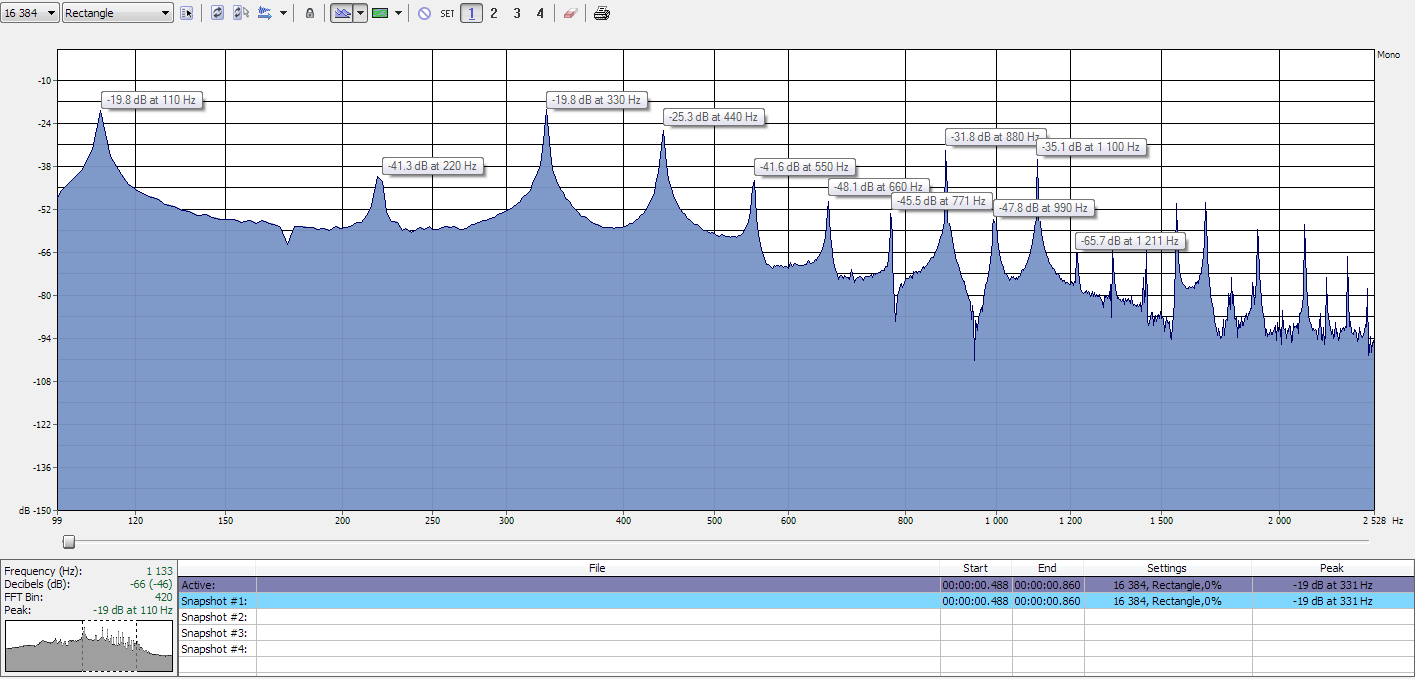# Analysis of a guitar string

What is a string and how does it vibrate? The picture below (Fig 3) is a model on how a string vibrates. At half the lenght we have the same note but an octave higher (the 12th fret) and is called the second harmonic on the open string. At those nodes we can find the harmonics of the string, also called overtones. For example a lengt of a string is 64 cm. The 2:nd harmonic would be at 32 cm, the 3:rd at 21.33 cm, and so on.

Fig 3The easiest ways to understand harmonics is to use flageolets. At the seventh fret we have the third harmonic (one third of the open strings length). The same harmonic can also be reproduced higher up on the neck at the 19th fret. At the fifth fret we find the fourth harmonic.

Harmonics is a factor of 2 as you can see in Fig 3. So what does this means frequency-wise?

## Using the A string as an example

Spectrum analysis of a guitar string at A. To my ears its fundamental frequency is at 220 Hz.

(Oddly enough ,when doing a FFT analysis, the fundamental frequency of the A string turns out to be 110 Hz. You can check it out in Analyze Instrument Frequencies)

### Harmonics of the A string in frequency

Fig 4Using the model in Fig 3 and looking at the peaks (overtones) in the FFT analysis in Fig 4. The model shows us that:

110 + 110 = 220 Hz, 110 + 110 + 110 = 320 Hz

Written as formula

Nth Harmonic Frequency = (Fundamental Frequency) x Nth harmonic
or exemplified with
110 = 110 x Nth harmonic

Overtones (harmonics) in the A-string

1. 110 Hz
2. 220 Hz
3. 330 Hz
4. 440 Hz
5. 550 Hz
6. 660 Hz
7. 770 Hz
8. 880 Hz
9. 990 Hz
10. 1100 Hz
11. 1210 Hz
12. to inf

As we can see in the spectrogram there are a lot of overtones in a vibrating string.

Go and have a look at Frequency Analysis of Different Instruments, a real time FFT JavaScript analyser where you can see the frequency response of different instruments.

|•|